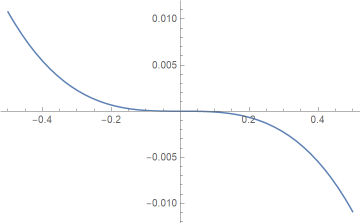# Mentally computing e^x

A few days ago I wrote about how to estimate 10x. This is an analogous post for

exp(x) = ex.

We will assume -0.5 ≤ x ≤ 0.5. You can bootstrap your way from there to other values of x. For example,

exp(1.3) = exp(1 + 0.3) = e exp(0.3)

and

exp(0.8) = exp(1 – 0.2) = e / exp(0.2).

I showed here that

loge(x)≈ (2x – 2)/(x + 1)

for x between exp(-0.5) and exp(0.5).

Inverting both sides of the approximation shows

exp(x) ≈ (2 + x)/(2 – x)

for x between -0.5 and 0.5.

The maximum relative error in this approximation is less than 1.1% and occurs at x = 0.5. For x closer to the middle of the interval [-0.5, 0.5] the relative error is much smaller.

Here’s a plot of the relative error.This was produced with the following Mathematica code.

```    re[x_] := (Exp[x] - (2 + x)/(2 - x))/Exp[x]
Plot[re[x], {x, -0.5, 0.5}]
```

Update: The approximations from this post and several similar posts are all consolidated here.

## 2 thoughts on “Mentally computing e^x”

1. Diethard Michaelis

Typo in log_e formula: 2nd – must be a +
(and (x) argument is missing).

2. Thanks!